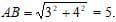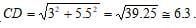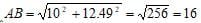GMAT  >  Test: Quantitative Aptitude- 1

# Test: Quantitative Aptitude- 1

Test Description

## 30 Questions MCQ Test Quantitative for GMAT | Test: Quantitative Aptitude- 1

Test: Quantitative Aptitude- 1 for GMAT 2022 is part of Quantitative for GMAT preparation. The Test: Quantitative Aptitude- 1 questions and answers have been prepared according to the GMAT exam syllabus.The Test: Quantitative Aptitude- 1 MCQs are made for GMAT 2022 Exam. Find important definitions, questions, notes, meanings, examples, exercises, MCQs and online tests for Test: Quantitative Aptitude- 1 below.
Solutions of Test: Quantitative Aptitude- 1 questions in English are available as part of our Quantitative for GMAT for GMAT & Test: Quantitative Aptitude- 1 solutions in Hindi for Quantitative for GMAT course. Download more important topics, notes, lectures and mock test series for GMAT Exam by signing up for free. Attempt Test: Quantitative Aptitude- 1 | 30 questions in 60 minutes | Mock test for GMAT preparation | Free important questions MCQ to study Quantitative for GMAT for GMAT Exam | Download free PDF with solutions
 1 Crore+ students have signed up on EduRev. Have you?
Test: Quantitative Aptitude- 1 - Question 1

### 15 Java programmers, working in a constant pace, finish a web page in 3 days. If after one day, 9 programmers quit, how many more days are needed to finish the remainder of the job?

Detailed Solution for Test: Quantitative Aptitude- 1 - Question 1

The total working days for finishing a web page are (15 x 3) 45. If after one day 9 programmers quit, only 15 working days are done and the rest of the programmers (6)

Need to finish (45 – 15) 30 days of work. It will take them 5 more days.

Test: Quantitative Aptitude- 1 - Question 2

### Two carpenters, working in the same pace, can build 2 desks in two hours and a half. How many desks can 4 carpenters build in 4 hours?

Detailed Solution for Test: Quantitative Aptitude- 1 - Question 2

⇒ 2 carpenters build 2 desks in 2.5 hours

⇒ 4 carpenters build 4 desks in 2.5 hours

⇒ In 4 hours there are (4/2.5 = 1.6) time units. And (4 x 1.6) is 6.4 desks.

Test: Quantitative Aptitude- 1 - Question 3

### There are 40 students in a classroom, 9/20 of them are boys and 4/5 of them are right-handed. How many right-handed boys are there in the classroom?

Detailed Solution for Test: Quantitative Aptitude- 1 - Question 3

Given that there are 18 boys (9/20*40=18) and 22 girls in the class. Also we know that out of 40 students 32 are right-handed (4/5*40=32).

Maximum number of right-handed boys possible is if ALL 18 boys are right-handed;
Minimum number of right-handed boys possible is if ALL 22 girls are right-handed, so in this case 32-22=10 boys would be right-handed.

Test: Quantitative Aptitude- 1 - Question 4

In Jonathan’s pen there are 300 sheep’s. 5/6 of the sheep’s are white, 2/3 of the sheep’s have soft wool. What can’t be the number of white sheep’s that also have soft wool in the pen?​

Detailed Solution for Test: Quantitative Aptitude- 1 - Question 4

There are (5/6 x 300 = 250) white sheep’s.
There are (2/3 x 300 = 200) soft woolen sheep’s.
The maximum overlap is the size of the smallest among the groups, thus 200. The minimum overlap is (250 + 200 – 300 = 150).
Therefore the number of sheep’s can be somewhere between 150 and 200.

Test: Quantitative Aptitude- 1 - Question 5

Ross has 40 shirts, ¾ of the shirts are green and 1/10 is without buttons.

Therefore Ross has between ___ and ___ shirts with buttons that are not green.

Detailed Solution for Test: Quantitative Aptitude- 1 - Question 5

Notice that the groups that we are looking for a overlapping are the not-green shirts and the buttoned ones. The not-green shirts are a quarter of 40, 10 shirts.
The shirts with buttons are (9/10 x 40 = 36).
The maximum overlapping is the size of the smallest group: 10.
The minimum overlapping is: 36 + 10 – 40 = 6.
Therefore A is the answer.

Test: Quantitative Aptitude- 1 - Question 6

In the Kan film festival, 50 movies were presented. 3/5 of the movies are action movies and 4/5 is science fiction movies. How many of the movies were science fiction action movies?

Detailed Solution for Test: Quantitative Aptitude- 1 - Question 6

There were (3/5 x 50 = 30) action movies.
There were (4/5 x 50 = 40) science fiction movies.
Exact overlapping is calculated by minimum overlapping method.
Therefore there are (40 + 30 – 50 = 20) movies that belong to both categories.

Test: Quantitative Aptitude- 1 - Question 7

There are 200 cats in Cat-City. Out of the 200, 70 are street cats and the rest are domestic cats. 110 cats are gray, 30 out of the gray cats are street ones. How many domestic cats are there which are not gray in Cat-City?

Detailed Solution for Test: Quantitative Aptitude- 1 - Question 7

Out of 200 cats, 130 are domestic ones. Out of 110 gray cats, 30 are street cats therefore 80 are grey and domestic ones.
Altogether there are 130 domestic cats, 80 are grey so (130 – 80) = 50 are not grey.

Test: Quantitative Aptitude- 1 - Question 8

Chandler is building a fence in the following method: He grounds 10 poles, each 10 Cm thick, in 1 meter spaces from each other. He then connects the poles with a barbed wire. What is the total length of the fence?

Detailed Solution for Test: Quantitative Aptitude- 1 - Question 8

The total width of the poles is (10 x 0.1 = 1) meter.
There are 9 spaces between the poles, each 1 meter, so it’s another 9 meters.
The total length is (1 + 9 = 10) meters.

Test: Quantitative Aptitude- 1 - Question 9

In a psychology school the grade of the students is determined by the following method: At the end of the first year the grade equals to twice the age of the student. From then on, the grade is determined by twice the age of the student plus half of his grade from the previous year. If Joey’s grade at the end of the first year is 40, what will be his grade at the end of the third year?

Detailed Solution for Test: Quantitative Aptitude- 1 - Question 9

From the grade 40 at the end of the first year we learn that his age is 20.
At the end of the second year, he will be 21 and his grade will be
(21 x 2 + ½ x 40 = 62).
At the end of the third year, he will be 22 and his grade will be (22 x 2 + ½ x 62 = 75).

Test: Quantitative Aptitude- 1 - Question 10

What is the sum of all the even numbers bigger than (-10) and smaller than 12?

Detailed Solution for Test: Quantitative Aptitude- 1 - Question 10

This is a series of numbers with a constant spacing between them.
The first number is (-8) and the last is (10), there are 10 numbers altogether.
The formula for such a series is: ((-8 + 10) x 10)/2 = 10.
The second way to answer such a question is to write the numbers and add them

Test: Quantitative Aptitude- 1 - Question 11

The value of an “Aerosoul” stock changes according to the following method:At the end of each month her value is doubled but due to commission the stock’s value is decreases by \$10. If the value at the beginning of January is \$A, what would be her value at the end of February?

Detailed Solution for Test: Quantitative Aptitude- 1 - Question 11

At the end of January her value is 2A – 10.
At the end of February her value is (2 x (2A – 10) – 10 = 4A – 30).

Test: Quantitative Aptitude- 1 - Question 12

An Ameba is an organic life form that divides into two Amebas each round hour. If at a certain round hour, two Amebas were placed in a jar, how many Amebas will be in the jar in N hours?

Detailed Solution for Test: Quantitative Aptitude- 1 - Question 12

Let’s find the number of Amebas in the first hours.
After one hour (N=1) there will be 4 Amebas.
After two hours (N=2) there will be 8 Amebas.
After three hours (N=3) there will be 16 amebas.
Therefore the formula that fits this series is 2N+1.

Test: Quantitative Aptitude- 1 - Question 13

Alfa, Beta and Gamma are inner angles in a triangle. If Alfa = Beta + Gamma, what can’t be the size of Beta?

Detailed Solution for Test: Quantitative Aptitude- 1 - Question 13

If Beta is 90 degrees than Alfa is bigger than 90 and the sum of the angles in the triangle will be bigger than 180 degrees.

Test: Quantitative Aptitude- 1 - Question 14

In a triangle, one side is 6 Cm and another side is 9 Cm. which of the following can be the perimeter of the triangle?

Detailed Solution for Test: Quantitative Aptitude- 1 - Question 14

The third side of the triangle is larger than 3 (The difference between the other two) and smaller than 15 ( The sum of the other two).
The perimeter is between (6+9+3 = 18) and (6+9+15 = 30). The only answer that is in this range is B.

Test: Quantitative Aptitude- 1 - Question 15

To which of the following shapes the area can’t be calculated if the perimeter is given?

Detailed Solution for Test: Quantitative Aptitude- 1 - Question 15

The perimeter of a rectangle is 2a + 2b. In order to calculate the area we need to know the multiplication of a x b.

Test: Quantitative Aptitude- 1 - Question 16

A and B are two circles. The radius of A is twice as large as the diameter of B. What is the ratio between the areas of the circles?

Detailed Solution for Test: Quantitative Aptitude- 1 - Question 16

The radius of circle A is 4 times larger than the radius of circle B. The area of a circle is a function of the radius squared, therefore the area of radius A is 16 times bigger.

Test: Quantitative Aptitude- 1 - Question 17

A, B, C, D and E are 5 consecutive points on a straight line. If BC = 2CD, DE = 4, AB = 5 and AC = 11, what is the length of AE?

Detailed Solution for Test: Quantitative Aptitude- 1 - Question 17

First, draw the line and the points.
In order to find the length of AE, find the length of CD and BC first.
BC = AC – AB = 11 – 5 = 6.
BC = 2CD à CD = 3.
AE = 5 + 6 + 3 + 4 = 18.

Test: Quantitative Aptitude- 1 - Question 18

In a rectangular axis system, what is the distance between the followingpoints: A(3,2) and B(7,5) ?

Detailed Solution for Test: Quantitative Aptitude- 1 - Question 18

First, draw a rectangular axis system and mark the two points.
The easiest way to find the distance between them is to draw a triangle, where the line AB is the hypotenuse. You can see that the length of one side of the triangle is (5-2=3) and the other side is (7-3=4). The length of the line AB is received with the help Of the Pythagoras principle:Test: Quantitative Aptitude- 1 - Question 19

In a rectangular axis system, what is approximate distance between the following  points: C(1,2.5) and D(6.5,5.5) ?

Detailed Solution for Test: Quantitative Aptitude- 1 - Question 19

First, draw a rectangular axis system and mark the two points.
The easiest way to find the distance between them is to draw a triangle, where the line CD is the hypotenuse. You can see that the length of one side of the triangle is (5.5 - 2.5 = 3) and the other side is (6.5 – 1 = 5.5). The length of the line CD is received with the help Of the Pythagoras principle:Test: Quantitative Aptitude- 1 - Question 20

In a rectangular axis system, what is the distance between the following points: A(24.4,30) and B(34.4,42.49) ?

Detailed Solution for Test: Quantitative Aptitude- 1 - Question 20

First, draw a rectangular axis system and mark the two points.

The easiest way to find the distance between them is to draw a triangle, where the line AB is the hypotenuse. You can see that the length of one side of the triangle is

(34.4 – 24.4 = 10) and the other side is (42.49 – 30 = 12.49). The length of the line AB is received with the help of the Pythagoras principle:Test: Quantitative Aptitude- 1 - Question 21

In a rectangular axis system, what is the area of a parallelogram with the coordinates: (5,7), (12,7), (2,3), (9,3) ?

Detailed Solution for Test: Quantitative Aptitude- 1 - Question 21

First, draw the axis system and mark the 4 points. Connect the points to get a parallelogram. The area is calculated by the multiplication of one on of the bases and the height. The height is (7 – 3 = 4), the length of the base is (9 – 2 = 7).
The area is 4 x 7 = 28.

Test: Quantitative Aptitude- 1 - Question 22

If the radius of a cylinder is doubled and so is the height, what is the new volume of the cylinder divided by the old one?

Detailed Solution for Test: Quantitative Aptitude- 1 - Question 22

The volume of a cylinder is (pie x R2) x (height of cylinder).
The new volume is (4 x 2 = 8) bigger.

Test: Quantitative Aptitude- 1 - Question 23

If the radius of a cylinder is doubled and so is the height, how much bigger is the new lateral surface area (with out the bases)?

Detailed Solution for Test: Quantitative Aptitude- 1 - Question 23

The best answer is D.
The lateral surface area of a cylinder is (2 x pie x R) x (height of cylinder).
The new lateral surface area is (2 x pie x 2R) x (double the height) = 4 times bigger.

Test: Quantitative Aptitude- 1 - Question 24

If  X ~ Y = X2 + XY, then what is the value of  -1 ~ 2 ?

Detailed Solution for Test: Quantitative Aptitude- 1 - Question 24

The best answer is B.-1 ~ 2 = (-1)2 + (-1)2 = -1.

Test: Quantitative Aptitude- 1 - Question 25

For every X, the action [X] is defined in the following matter: [X] is the greatest integer that is smaller or equal to X. For example: [8.9] = 8.

What is the value of [6.5] x [2/3] +  x 7.2 + [8.4] – 6.6 ?

Detailed Solution for Test: Quantitative Aptitude- 1 - Question 25

The best answer is A.

[6.5] x [2/3] +  x 7.2 + [8.4] – 6.6 = 6 x 0 + 2 x 7.2 + 8 - 6.6 = 15.8.

Test: Quantitative Aptitude- 1 - Question 26

If  (1 < A < 3 < B), then which of the following expressions is the largest?

Detailed Solution for Test: Quantitative Aptitude- 1 - Question 26

The best answer is A.
Try some numbers and check the answers. A=2, B=4.
(a) 6/1 = 6
(b) 2/3
(c) 1/2
(d) 2
(e) 2

Test: Quantitative Aptitude- 1 - Question 27

Which of the following fractions is the smallest?

Detailed Solution for Test: Quantitative Aptitude- 1 - Question 27

The best answer is A
.Compare all of the answers to
(a) 3/10.
(b) 3/10 x 2 = 6/20 which is smaller than 6/19.
(c) 3/10 is smaller.
(d) 3/10 = 9/30, and this is smaller than 11/30.
(e) 3/10 = 12/40 and that is smaller than 12/31.
The smallest fraction is A.

Test: Quantitative Aptitude- 1 - Question 28

Which of the following fractions is the largest?

Detailed Solution for Test: Quantitative Aptitude- 1 - Question 28

The best answer is C.
Lets compare all the answers to 2/7, unless we find a larger fraction.
(b) 2/3 is larger than 2/7. For now, this is the right answer.
(c)  2/3 is also 6/9 and that is smaller than 7/9. For now this is the right answer.
(d) 7/9 is bigger than 7/12
​(e) Bring this answer and (c) to a common denominator.7/9 = 35/45  and  3/5 = 27/45.
7/9 is the largest fraction.

Test: Quantitative Aptitude- 1 - Question 29

If A2 + B2 = 15 and AB = 10, what is the value of the expression (A – B)2  + (A + B)2 ?

Detailed Solution for Test: Quantitative Aptitude- 1 - Question 29

The best answer is C.
(A – B)2  + (A + B)2 = A2 – 2AB + B2 + A2 + 2AB + B2 = 2(A2 + B2) =  30.

Test: Quantitative Aptitude- 1 - Question 30

If A  and B are positive integers, which of the following expressions is not an integer for certain?

Detailed Solution for Test: Quantitative Aptitude- 1 - Question 30

The best answer is D.
All the answers besides D are numbers after some simplification.Answer D = (A + B)/(A+B)2 = 1/(A+B), and this is a fraction of a number.

## Quantitative for GMAT

71 videos|83 docs|147 tests
 Use Code STAYHOME200 and get INR 200 additional OFF Use Coupon Code
Information about Test: Quantitative Aptitude- 1 Page
In this test you can find the Exam questions for Test: Quantitative Aptitude- 1 solved & explained in the simplest way possible. Besides giving Questions and answers for Test: Quantitative Aptitude- 1, EduRev gives you an ample number of Online tests for practice

## Quantitative for GMAT

71 videos|83 docs|147 tests